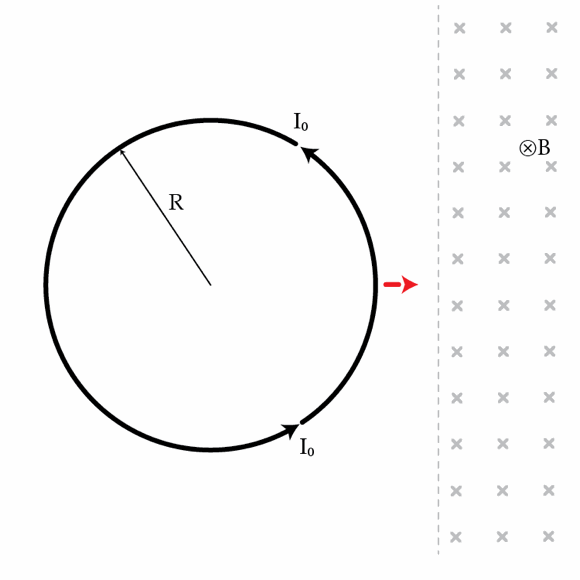# Work done on a superconductor

A superconducting (zero resistance) ring of radius $R=2~\mbox{cm}$ and self inductance $L=0.5~\mbox{nH}$ is located in a region of zero magnetic field. A current $I_{0}=0.1~\mbox{A}$ circulates around the ring as shown in the figure below. What minimum work in Joules must be done on the ring to fully immerse it in a region with a uniform magnetic field $B=0.1~\mbox{T}$ (directed into the page)?×